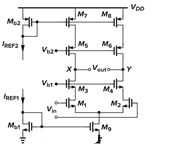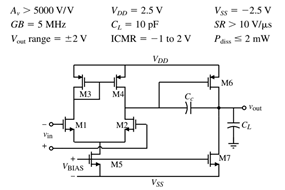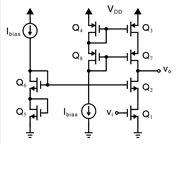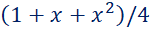# Department of Electronics Engineering

## Important Laboratories

Dept. data last updated on :27/09/2022

## List of Experiments

 1 Amplitude Modulation (AM) Generate AM wave for the given specifications and plot its amplitude spectrum. Plot the modulation characteristic of the give AM modulator. 2 Envelope Detection Perform envelope detection of the specified AM wave. Observe the effect of parameter variations of AM wave on the envelope detector. 3 Synchronous Detection Perform synchronous detection of the specified AM wave. Analyze the effect of phase and frequency error on synchronous detection. 4 Frequency Modulation (FM) Perform frequency modulation using VCO and plot its amplitude spectrum. Observe the effect of modulation index on the spectrum of FM wave. 5 FM Demodulation Perform FM demodulation for different values of modulation index in the absence of noise. 6 Uniform and Non-uniform Quantizer 7 Matched Filter Study of matched filter to verify it as an optimum detector in the presence of AWGN. 8 Sampling and Reconstruction Study and verify Nyquist sampling theorem.

## Venue: Communication Lab

List of Experiments
 1.Record your own speech signal (say “hello”) using single channel with a sampling frequency of 16 kHz. Draw the power spectrum of the signal. Quantize the signal and generate a bit sequence using a uniform quantizer of 16 levels. 2.Represent the above sequence (first 20 bits only) into a waveform using polar NRZ signaling. Use rectangular pulse shaping. 3.Plot the quantization errors of the signal samples obtained in Exp. 1. Reconstruct the signal and observe the quality degradation in the reconstructed signal. 4.Generate a 16-QAM signal (assume carrier frequency of 500 kHz) using a rectangular constellation. Plot the QAM signal obtained corresponding to the waveform obtained from Exp. 2. Generate an eye pattern of the bit sequence obtained in Exp. 1 for a noiseless channel which has an ideal lowpass frequency characteristics of bandwidth 20 kHz. Determine (a) distortion (b) time jitter (c) noise margin (d) best sampling time, and (e) sensitivity to timing error. For a given H matrix, find G matrix of a (7,4) block code. For a given data word generate a corresponding codeword. Also write a program for syndrome decoding, in which all error patterns of 1 bit error are corrected. Now obtain (8, 4) code and show that all 2 bit errors are detectable by this code, (i) Use the bit sequence of Exp. 1 and pass it through a AWGN channel. Plot the BER curve by increasing SNR from 5 to 20 dB. Now apply channel coding of Exp. 6 and repeat (i).

## Course: VLSI and Signal Processing Lab (ELC4910)

Venue: Computer Lab

List of Experiments - VLSI Part

 Lab-1: Write a behavioural Verilog HDL code to implement a four-bit full adder/subtractor. The user decides type of operation by a control input. When the control input is at logic level 1, subtraction will be done. When the control input is at logic level 0, addition is done. Write a test-bench to verify the operation of the designed adder/subtractor. Lab-2: Write a behavioural Verilog HDL code to implement a JK flip-flop for following cases: (a) Positive edge triggered JK flip-flop with active low asynchronous 'Clear' and 'Preset' inputs. (b) Negative edge triggered JK flip-flop with active low synchronous 'Clear' and 'Preset' inputs. Write a test-bench to verify the operation of both the flip-flops. Lab-3: Implement a digital lock in Verilog HDL that will accept a specific bit sequence “110100” through an input button “b_in” serially in synchronism with the negative edge of an input clock "clk" and will generate an “unlock” signal “1” as output. For any other bit sequence the “unlock” signal will remain at logic “0”. An active low “clear” signal is used to asynchronously reset the lock in its initial/default state. Lab-4: Write a Verilog HDL code for a Mealy type FSM that has an input 'W' and an output 'Z'. The machine has to generate Z = 1 when the previous four values of 'W' were 1001 or 1111; otherwise, Z = 0. Overlapping input patterns are allowed. An example of the desired behavior is: W : 010111100110011111 Z  : 000000100100010011 Write a test-bench to verify the operation of the designed FSM.

## Venue: Signal Processing Lab

List of Experiments – DSP Part

The following all four experiments are to be performed:

1. MATLAB-based linear and circular convolution. Write MATLAB codes for performing linear and circular convolution using your own function and in-build function. Compare the results in both cases and measure the time elapsed in both cases.

2. MATLAB-based Image format conversion, PSNR calculation, and 2-D filtering operations.

Write and execute a MATLAB code to

(a) RGB image into a grayscale image.

(b) Perform spatial domain low pass filtering of an image and evaluate the difference between the filtered image and the original image using a quantitative metric called Peak signal to noise ratio (PSNR).

(c) Perform spatial domain high pass filtering of the image.

3. N-point DFT of a given sequence on the DSP kit. Write a C program to calculate the N-point DFT of the given sequence using the decimation-in-time FFT algorithm. Compile your code on TMS320EVM6747 DSK using code composer studio. Take any arbitrary sequence of length N and obtain the DFT output. Also, obtain the DFT output for the same sequence using MATLAB and verify both results. Also, repeat the above experiment with decimation in the frequency FFT algorithm.

4. Design and implementation of FIR bandpass filter using C programing language to be executed on TMS320C6416 DSP kit. Design N-tap linear phase bandpass FIR filter using windowing method for a sampling rate of fs kHz. Compile the code on TMS320C6416 DSK using code composer studio. The passband extend from f1 kHz to f2 kHz. Store the filter tap coefficients and store it using MATLAB.

## Course: Electronics lab - III (ELC3910)

Venue: Computer Lab

List of experiments

 Note: All simulations are to be perform using PSPICE Rotor-I 1(i) Determine the currents and voltages for a given resistive circuit.   (ii)Plot the transient and frequency response of RC circuit for different values of R. 2(i) Plot the I-V characteristics of a semiconductor diode for different operating temperatures.    (ii)Transient response of Clipper and Clamper circuits 3) Study of CE amplifier with different types of loads. Determine the small signal voltage gain, input and output resistance in each case. 4) Transfer characteristics of Push-pull amplifier with THD calculation Rotor-II 5(i) Sallen and Key filter   (ii) Wien bridge oscillator design for a given frequency 6(i) Common Source amplifier design   (ii)CMOS logic gates 7) Design and test of a Differential amplifier 8) Design and test of a Transconductance amplifier Rotor-III 9) Mini Project (Cascode / High gain differential amplifier with Current mirror biasing)

## Course: Laboratory-I (ELC6911)

Venue: Computer Lab

List of experiments

 Lab-1: Design a common source amplifier with PMOS/NMOS diode connected load using the 0.18 um CMOS device parameters and assuming a supply voltage of 1.8V. The amplifiers should have a maximum power consumption of 0.18 mW and a gain of at least 2 V/V. Channel length of all the transistors are L=300 nm. Lab-2: Design a common-source amplifier with NMOS current source load and PMOS driver using the 0.18-μm CMOS device parameters and assuming a supply voltage of 1.8 V. The amplifier should have a maximum power consumption of 1 mW, and a gain of at least 20 V/V. Ensure all transistors have an overdrive voltage of 200mV and gate length L=0.25 um. Lab-3: Design the cascode amplifier topology, shown in figure 1, using the 0.18-μm CMOS device parameters and assuming a supply voltage of 1.8 V. The amplifier should have a maximum power consumption of 0.54 mW, and a gain of at least 200 V/V. All the transistors have gate length L=0.25 um. Lab-4: Design a differential amplifier with current source/current mirror load in 0.18u technology with a power budget of 2mW and load capacitance of 1pF for a suitable specification in terms of differential gain, differential voltage swing and bandwidth. Also design an appropriate biasing network to generate Iss and Vb. Lab-5: Design a fully differential telescopic amplifier shown in figure2 with the following specifications: VDD = 3 V, peak-to-peak differential output swing = 3 V, maximum power dissipation = 10 mW and minimum voltage gain = 2000. Use 0.35 um technology parameters with the length of all the transistors fixed at 0.5 um. Lab-6: Using 0.35 um device parameters, design the amplifier circuit shown in figure 3 to meet the given specifications with a phase margin of 60°. Fix the channel length of all transistors at 1 um.Figure 1                                           Figure 3                                       Figure 2

## Course:Lab-II (ELC6912)

Venue: Computer Lab

List of Experiments

A. Implement and simulate the following hardware using Verilog HDL. Also synthesize the same.

1. Full Adder, Multiplexer(2x1), Two-Bit Comparator, and Decoder (2x4)

2. D-latch,

3. D-flip flop,

4. 4-bit synchronous up counter,

5. 4-bit shift register using d-flip flop.

6. Given finite state machine.

7. Synchronous FIFO

8. Single port Random Access Memory (RAM).

B. Project: equivalent to 3 lab sessions.

## Venue: Digital Systems and Microprocessor Lab

List of Experiments

 Phase-I1.Write a program using 8085 kit for finding Smallest, Largest, Second smallest, Second largest number in an array of N numbers2. Write a program using 8085 kit to find the value of expression, where x is an 8 bit number.3. Write a program using 8085 kit to arrange N numbers in (a) Ascending order; (b) Descending order using Bubble sort and Selection sort algorithm.4. Write a program using 8085 kit for:       Multiplication of Two 8-bit numbers (using Shift/Add method)       Divide a 16-bit number by an 8-bit number using the rotation method. Phase-II Write an 8085 program to generate square, triangular, sawtooth, and complex waveform using a DAC card.Write a program to interface an 8-bit ADC 0808 with an 8085 microprocessor. Phase-III Project: Demonstrate any two ideas using Arduino microcontroller (in a group of 3 students).

## Venue: Digital Systems and Microprocessor Lab

List of Experiments

 1 a. Introduction to the digital laboratory equipment used, report and evaluation. b. To verify the Truth Tables of basic logic gates. 2 Plot the transfer characteristics of the CMOS inverter by varying the input voltage from 0 V to 5 V with the step of 0.2V and measuring the output voltages. Also, verify the truth table of CMOS NAND and NOR gates. 3 To implement and verify 4 To test a 4-bit programmable adder/ subtractor 5 To test an 8 to 1 line multiplexer and use it to implement a given 4-variable logical function. 6 To verify the truth table of the following types of Latches and Flip Flops with control inputs(a) R-S (b) D (c) T (d) J-K 7 To design and Implement the circuit of MOD-8 asynchronous and MOD-6 synchronous counters using J-K flip-flops. 8 Study and verify the load, shift and rotate operation of a 4-bit shift register. 9 To design and test a 4-bit R-2R ladder type DAC.

## Venue: Electronics Lab

List of Experiments

 1 To study and test various components and measuring instruments used in different experiments. 2 (a). Plot the forward and reverse characteristics of a semiconductor diode.              (b). Plot the characteristics of the Zener diode in the reverse breakdown region. 3 Design and test an RC Passive Network for a given phase shift Φ at a given frequency f-------. 4 Design and implement a circuit to integrate and differentiate sine and square waveforms. 5 Design and implement an OPAMP-based inverting and non-inverting amplifier circuit for a gain of-------. 6 Design and implement a full-wave rectifier circuit with and without a filter. 7 To plot the input and output characteristics of NPN BJT in Common Emitter Configuration. 8 To design and test a Wein’s bridge oscillator for a given frequency of --------kHz.

## Venue: Electronics Lab

List of Experiments

 1 To study and test various components and measuring instruments used in different experiments. 2 To plot the forward and reverse bias characteristics of semiconductor and Zener diodes. Also, measure the cut-in/breakdown voltages of respective diodes. 3 To trace the output of a full-wave rectifier with and without a filter. 4 To plot the input and output characteristics of a given BJT. 5 To design and test op-amp based inverting and non-inverting amplifiers. Part 1: To verify the Truth Tables of basic logic gates.           Switches A, B, C are on.           Switches A and B are on but switch C is off.           Switches A and C are on but switch B is off.           Switches C and B are on but switch A is off.Obtain a truth table for this situation and hence a Boolean expression. Minimize this expression and implement the logic circuit using only NAND gates. 6 To implement and test a 4-bit programmable adder/subtracter. 7 To implement and test a 3-bit ripple counter. 8 To design and test a MOD-6 synchronous counter.

## Venue: Electronics Lab

List of Experiment

 To study and test various components and measuring instruments used in different experiments. 2. Plot the forward and reverse characteristics of a semiconductor diode. 3. To study the use of Zener diode as a voltage regulator. 4. To implement a Full Wave Rectifier (with and without filter) using diodes. 5. To Design and test a BJT based digital logic inverter. 6. To study the pulse response of an RC circuit. 7. To Design and implement an OPAMP based non-inverting amplifier circuit for a gain of _____. 8. To Design and implement an OPAMP based integrator circuit. 9. To study the diode based clipper circuit.

## Venue: Instrumentation and Control Lab

List of Experiments

 1 Determine the dynamic volt-amp characteristics of the given SC diode and Zener diode and determine cut-in voltage and forward resistance & Zener breakdown voltage. 2 Design the Schmitt trigger for a given Hysteresis voltage and draw its transfer characteristics. 3 Measure the frequency and the frequency deviation, with the help of Weins Bridge. 4 Calibrate the given pressure measuring system. 5 Draw a calibration curve of the given strain gauge. 6 To calibrate the given thermistor. 7 Draw the calibration curve of the given L.V.D.T. 8 Measure the frequency and frequency deviation with the help of an active bridge. 9 Determine the value of unknown capacitance using the RC phase shift Oscillator. 10 Calibrate the wattmeter using the phantom method of loading.

## Course: Lab-I (ELC6921)

Venue: Computer Lab

List of Experiments

 1. Write a program for the Huffman Coding to compress. 2. Generate a binary random sequence and represent the data in certain forms of line coding techniques. Test these formats in the presence of AWGN (for a given value of power spectral density). 3. Generate differentially coded baseband binary waveforms for the given data and show that if all the received bits are reversed, the transmitted data is detected without error. 4.Generate M-ary QAM signal and determine the performance over AWGN channel for (a) M = 4 (b) M = 16. 5. Study of the wireless sensor network using WSN kits. 6. Mini-Project in the area of communication and signal processing.

## Course: Lab-II (ELC6922)

Venue: Computer Lab

List of Experiments

 Module 1 Simulation and bit error rate performance evaluation of Digital Communication systems over fading channel in MATLAB/Octave/Scilab. Module 2 Polyphase implementation of filter bank in MATLAB/ Octave/ SciLab. Module 3 Image filtering in spatial/frequency domain and spectral analysis using MATLAB/Octave/Scilab. Module 4 Adaptive filter design and implementation using LMS algorithm for given specifications in MATLAB/Octave/SciLab.

## PHOTOGRAPHS OF FEW LABORATORIES OF THE DEPARTMENT